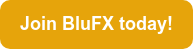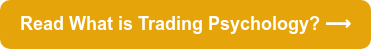Leverage is having the ability to control a large amount of money using a very small amount of your own and borrowing the rest.

Leverage essentially allows a small investment to be transformed into a larger profit. However, you will often hear leverage referred to as a double-edged sword - and it’s referred to this way for a reason! Leverage can be as risky as it can be beneficial for a trader - read on to find out how to navigate the risks involved.

Here’s our guide to leverage in forex…

Note: To understand leverage, it’s essential you understand margin. Need a refresher? Visit our What is Margin? blog.# What is leverage?

Leverage, as discussed above, is the ability to control a large amount of money using a small amount of your own money.

For example, in order to control a \$100,000 position, your broker will set aside \$1,000 from your account. So you can now control \$100,000 with \$1,000.

Leverage is expressed in ratios - so the leverage above is 100:1. This essentially means that you can control the full amount with 1% of it.

### How is leverage related to margin?

Margin and leverage are closely related. Based on the margin required by your broker, you can calculate the leverage you can wield. See below for how to use margin to calculate leverage.

### How do you calculate leverage?

To calculate leverage, you can perform the calculation below:

Leverage = total value of transaction / margin required

For example: \$10, 000 / \$1, 000 = 10 times or 10:1

You can also calculate leverage using the formula below if you know the margin requirement:

Leverage = 1 / margin

For example, as above, if the margin required is 0.01 (or 1%) for \$100,000, then your leverage is 100:1. The calculation is done as follows: 1/0.01= 100. This is then expressed as 100:1.

If the margin is 0.02 (or 2%), then leverage is 50:1. The calculation: 1/0.02 = 50. This is then expressed as 50:1.

### What is a common leverage ratio?

Here are some popular leverage and margin requirements offered by brokers:

 Margin required Leverage 5% 20:1 3% 33:1 2% 50:1 1% 100:1 0.50% 200:1

### Forex leverage for beginners: what you need to know

One of the most important things to understand about leverage if you’re new to trading is that it can be as risky as it can be beneficial because while it can amplify your gains, it can also amplify your losses. So the lesson here is that you need to understand how leverage works before beginning trading!

For example, if you placed \$1,000 for \$100,000 - and the investment rises in value to \$101,000, you would make \$1,000 profit (100% return on your initial margin, \$1,000). Great!

However, this is the catch: if the investment decreases in value by \$1,000, you would lose \$1,000. You can do the math: your losses would be 100%.

Essentially, the more leverage you use, the less room you have for the market to move before a margin call (you can read more about this on our Margin blog). This is why many successful traders use low leverage.

### What is the leverage at BluFX?

Many successful traders use low leverage, as there is significantly less risk involved. This is why, at BluFX, our leverage is 3:1, which we set up in line with our analysis of vast amounts of trader data.

Our leverage is set at this level so it allows traders with developed strategies to trade with low risk and consistent returns when using large amounts of trading capital. You can read more about this on our BluFX margin blog.